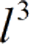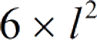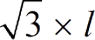Request a Tool

Cube Surface Area Calculator

Cube Surface Area calculator use to calculate digonal area, surface area and volume of a cube.

Volume
0
Surface Area
0
Diagonal Area
0

FormulaVolumeSurface AreaDiagonal Area

• l = Length of the Cube

Defination / Uses

A Cube is a three-dimensional shape with six square or rectangular sides. It is a three-dimensional solid object bounded by six square faces, facets or sides, where three of its sides meet at the vertex.

How to use cube surface area calcultor?

Its a single step procedure.

• Add the height of cube in its given field.

Thats it! You will get your answer in less than a second. Use upper given formulas in case of manual calculation

A cube has a volume and a surface area, just like any other 3D object. But, exactly, what is surface area? Sticking with our subject, a cube's surface area is the 2D surface that separates what's within from what's outside the cube. When you conceive of a square, the sides are what define where the square stops and where it begins. We need to go up one dimension in the case of a cube, so instead of a 1D line, we get a 2D surface. The precise phrase is "surface area," however for the cube, it's comparable to "the surface of a cube." We'll aim to use "surface area of a cube" as much as possible because it's more accurate, but you can use whatever you want. For quick calculation use our weetools. No sign-up, registration OR captcha is required to use this tool.